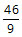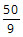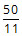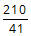# GMAT Practice Questions - Algebra

Must solve GMAT practice questions in algebra. The following topics are covered in the GMAT quant section from algebra:

1. Absolute Value
2. Functions

Click on the Problem Solving or the Data Sufficiency tab for practice questions. The topic from which the question has been selected and the concept tested are listed in the right column for each question. Detailed explanatory answers, solution videos and slide decks are also provided.

• 1
• If x is a positive integer such that (x-1)(x-3)(x-5)....(x-93) < 0, how many values can x take? A. 47
B. 23
C. 46
D. 21
E. 22
• Inequalities
• Positive & Negative numbers
• Odd & Even numbers
x can take 23 integer values.

Solution

• 2
• Working alone, A can complete a task in ‘a’ days and B in ‘b’ days. They take turns in doing the task with each working 2 days at a time. If A starts they finish the task in exactly 10 days. If B starts, they take half a day more. How long does it take to complete the task if they both work together? A.B.C.D.E.• 3
• If a, b, and c are not equal to zero, what is the difference between the maximum and minimum value of S? $$S = 1+\frac { \left| a \right| }{ a } +\frac {2 \left| b \right| }{ b } +\frac {3 \left| ab \right| }{ ab } -\frac {4 \left| c \right| }{ c } \\$ A. 12 B. 14 C. 22 D. 20 E. 18 • Problem Solving • Absolute Value • Correct AnswerChoice$E).
The difference between the maximum and minimum value is 18.

Solution

• 4
• If x and y are integers and |x - y| = 12, what is the minimum possible value of xy?
A. -12
B. -18
C. -24
D. -36
E. -48
• Problem Solving
• Absolute Value
The minimum possible value for xy is -36.

Solution

• 1
• Is x3 > x2 Statement 1: x > 0
Statement 2: x < 1

Solution

• 2
• If m and n are non-zero integers, is mn > nn? Statement 1: |m| = n
Statement 2: m < n
• Inequalities
• Exponents
• Absolute Value